Courses

# Heat Transfer in Boiling & Condensation - 4 Chemical Engineering Notes | EduRev

## Chemical Engineering : Heat Transfer in Boiling & Condensation - 4 Chemical Engineering Notes | EduRev

The document Heat Transfer in Boiling & Condensation - 4 Chemical Engineering Notes | EduRev is a part of the Chemical Engineering Course Heat Transfer.
All you need of Chemical Engineering at this link: Chemical Engineering

Illustration 6.1

Saturated steam at 70.14 kPa is condensing on a vertical tube 0.5 m long having an outer diameter of 2.5 cm and a surface temperature of 80oC. Calculate the average heat-transfer coefficient.

Solution 6.1

It is a problem of condensation on a vertical plate, thus eq.6.10b can be used,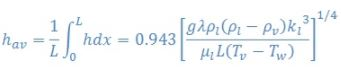where, different liquid and steam properties are evaluate at average film temperature,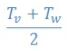Using steam table, the temperature of the steam corresponding to 70.14kPa pressure is 90oC. The average film temperature will then be the average of 80 oC and 90 oC and it comes out to be 85 oC,

Using given data the different properties can be found using steam table and other relevant tables given in the standard literature. The data is tabulates below at 85oC,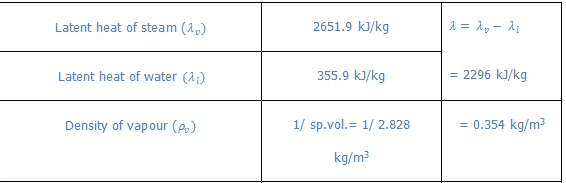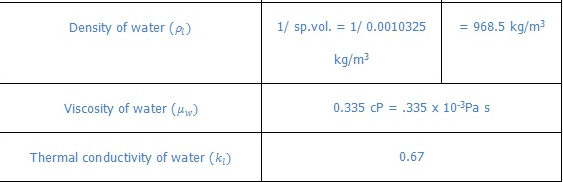On putting the above values in the above equation,

hav = 1205.2 W/m2 °C

6.5 Condensation for horizontal tube

6.5.1 Condensation outside horizontal tube or bank of tube
This type of condensation is very common especially for the shell and tube heat exchanger. In case of condensation outside the vertical array of horizontal tubes the condensate flows as a film along the cylindrical surface or it may drop down. In case of another tube below, the condensate film flows down from the bottom edge of the upper tube to the upper edge of the bottom tube. As it goes on to the lower tubes, the thickness of the condensate film increases. Some of the correlations are given below.

6.5.1.1 Condensation on a single horizontal tube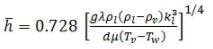(6.13)

6.5.1.2 Condensation on a vertical tube of N horizontal tubes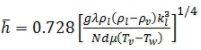(6.14)

6.5.1.3 Condensation inside a horizontal tube
Figure 6.5 shows the physical picture of the condensation inside a horizontal tube (like an open channel flow).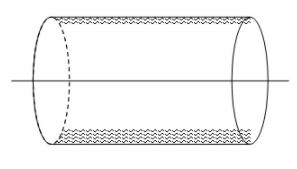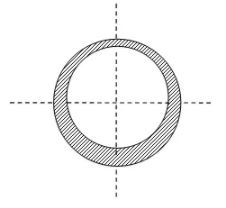Fig. 6.5: Film condensation inside a horizontal tube

Case 1: The length is small or the rate of condensation is low.

This situation will have small thickness of the flowing condensate layer at the bottom of the tube and the following coefficient can be used,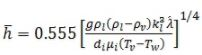(6.15)

where,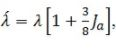and the vapour Reynolds number (Rev) should be less than 35,000. The Rev is calculated based on inlet condition of vapour and inside diameter of tube.

Case 2: The length is high or the rate of condensation is high.

In this the following relation can be used.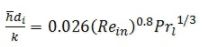(6.16)

where,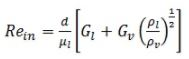(Condition: Rel >5000, Rev > 20,000)

where, Gl and Gv are the liquid and vapour mass velocities calculated on the basis of the cross-section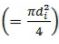of the tube.

6.6 Correlations for packed and fluidized bed

6.6.1 Packed bed
The heat transfer correlation for gas flow through a packed bed is given as,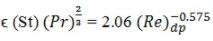(6.17)

Conditions to use eq.6.12 are,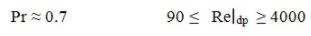where,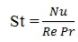is the Stanton number.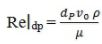is the particle Reynolds number

dp = Diameter or the effective diameter of a particle

v0 = Superficial fluid velocity. It is the velocity based on the cross-section of the bed).

∈ : Bed porosity or void fraction

∈ : 0.3 → 0.5

Theoretically :   ∈ = 0.69 for uniform shape

= 0.71 bed of cubes

= 0.79 bed of cylinder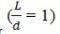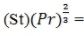Colburn factor

6.6.2 Fluidized bed

The heat transfer coefficient to or from particles in a fluidized bed can be estimated with the help of following correlation,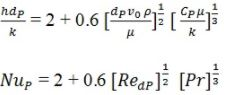(6.18 )

where, v0 is the superficial velocity.

Offer running on EduRev: Apply code STAYHOME200 to get INR 200 off on our premium plan EduRev Infinity!

## Heat Transfer

59 videos|70 docs|85 tests

,

,

,

,

,

,

,

,

,

,

,

,

,

,

,

,

,

,

,

,

,

;